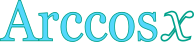## Inverse cosine calculator

This tool evaluates the inverse cosine of a number: arccos(x). The principal branch is evaluated, where the return values range between 0 and π. The domain of argument x is restricted to [-1,1].

 x = Result: arccos(x) = deg rad## Definitions

### General

The inverse cosine function, in modern notation written as arccos(x), gives the angle θ, so that:

Because the values of cosine function range between -1 and 1, the domain of argument x, in arccos function, is restricted to the same range: [-1,1]. Also, due to the periodical nature of the cosine function, there are many angles θ that can give the same cosine value (i.e. θ+2π, θ+4π, etc.). As a result, it is impossible to define a single inverse function, unless the range of the return values is restricted, so that a one-to-one relationship between θ and cosθ can be established. Therefore, multiple branches of the arccos function can be defined. Commonly, the desired range of θ values spans between 0 and π. The branch of arccos, in that case, is called the principal branch.

### Series

The arccos function can be defined in a Taylor series form, like this:

The above series is valid for |x|≤1. From the expanded form of the series, it can be seen that the higher terms become insignificant, for values of x close to zero, resulting in the following quite useful approximation:

### Properties

The derivative of the arccos function is:

The integral of the arccos function is given by:

The following properties are also valid for the arccos function: